# Volterra series

(diff) ← Older revision | Latest revision (diff) | Newer revision → (diff)

integro-power series

A series containing the powers of the unknown function under the integral sign. Letbe a continuous function in all variables in a cube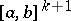and let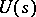be an arbitrary continuous function on. The expression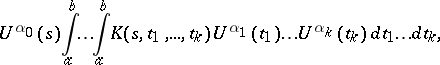where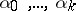are non-negative integers and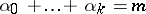, is called a Volterra term of degreein. Two Volterra terms of degreebelong to the same type if they differ only in their kernels. The finite sum of Volterra terms (of all types) of degreeis called a Volterra form of degreein the function. It is denoted by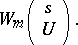Let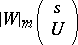denote the Volterra form in which the kernelis replaced by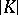, and let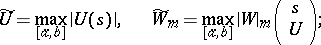thenThe expression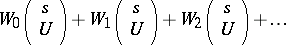is called a Volterra series. If the series of numbers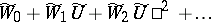converges, then the Volterra series is called regularly convergent. In this case the Volterra series converges absolutely and uniformly, and its sum is continuous on.

Analogously one introduces Volterra series in several functional arguments, and Volterra series in whichis replaced by some closed bounded set in a finite-dimensional Euclidean space. Volterra series are a particular case of the more general concept of an abstract power series.

How to Cite This Entry:
Volterra series. Encyclopedia of Mathematics. URL: http://encyclopediaofmath.org/index.php?title=Volterra_series&oldid=11667
This article was adapted from an original article by V.A. Trenogin (originator), which appeared in Encyclopedia of Mathematics - ISBN 1402006098. See original article